# Chapter 10: Figures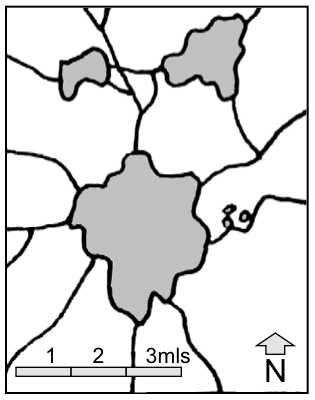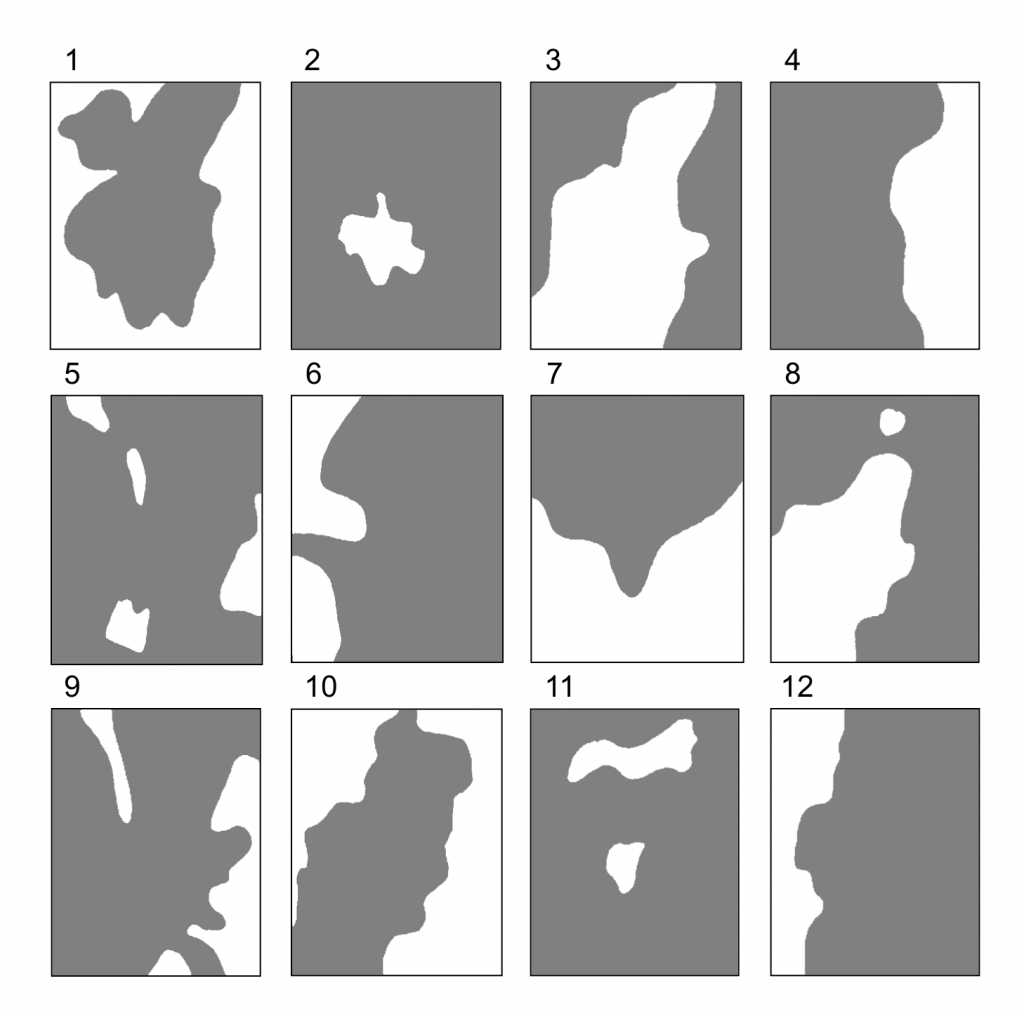Figure 10.1: Factors Represented as Partial Solutions to the Location Problem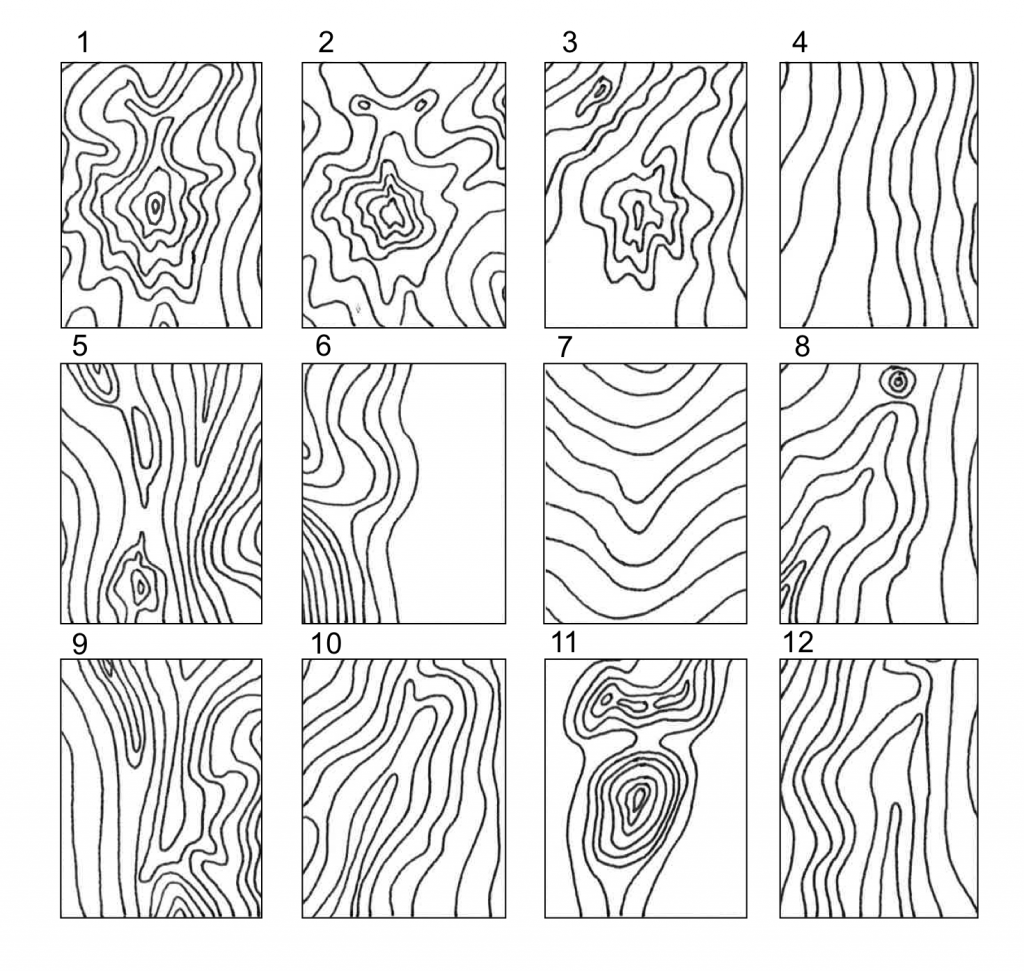Figure 10.2: Factors Represented as Desirability Surfaces for Best Locations

Figure 10.3: A Comparison of Different Linear Syntheses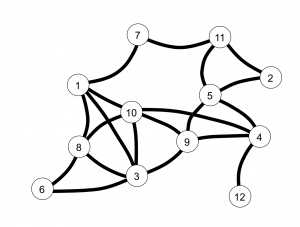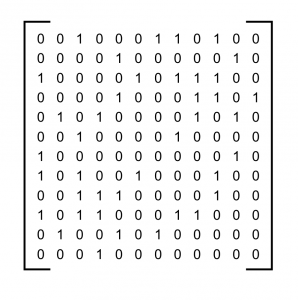Figure 10.4: The Graph of the Problem and Its Associated Adjacency Matrix

Figure 10.5: A Maximal Spanning Tree and Related Hierarchy

Figure 10.6: Hierarchical Structure Based on the Application of Ward’s Algorithm With the Without Contiguity Constraints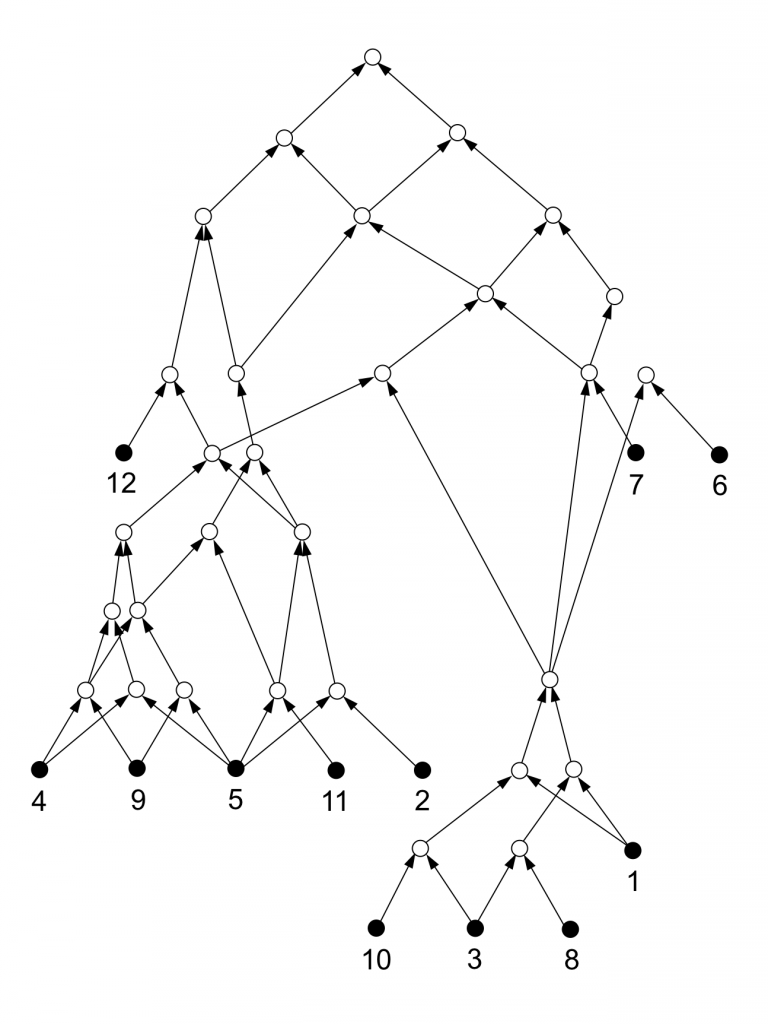Figure 10.7: The Resultant Lattice-Like Structure

Figure 10.8: A Comparison of the Syntheses Derived from the Hierarchical Methods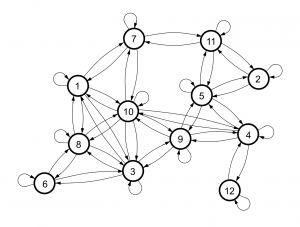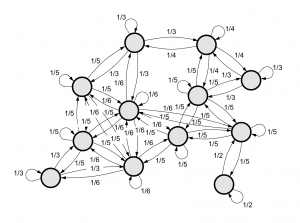Figure 10.9: The Power Structure as a Directed Graph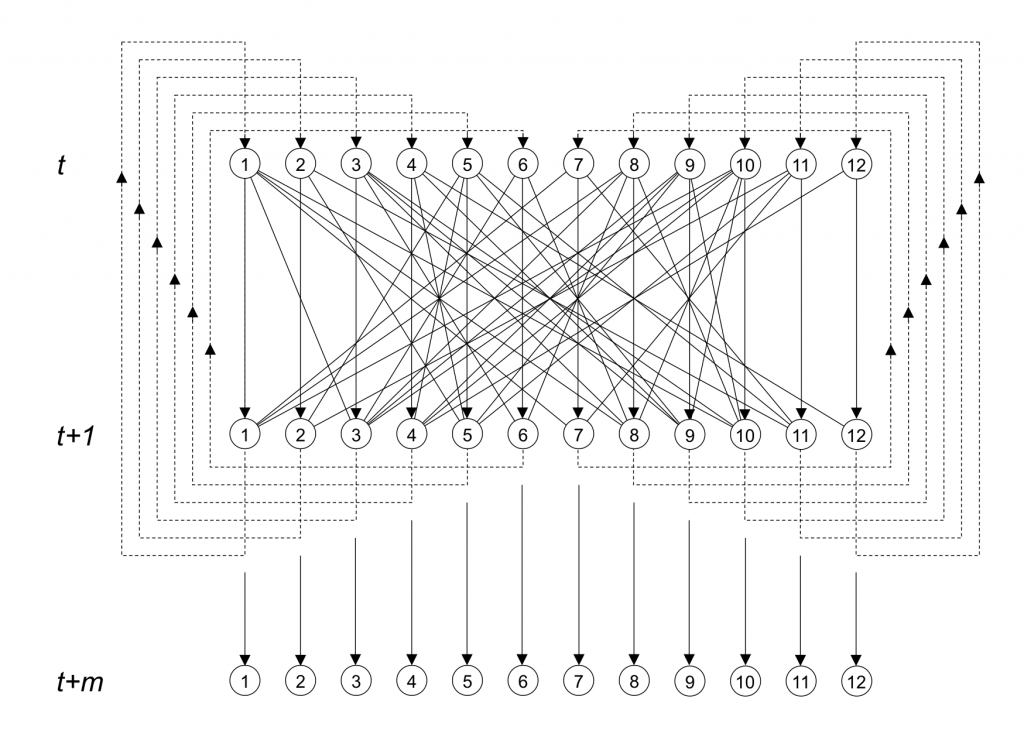Figure 10.10: The Markov Process as Sequential AveragingFigure 10.11: The Spatial Averaging in Constraint Map Form